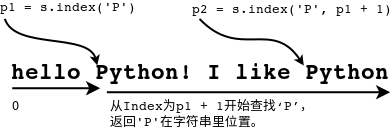# 10 字符串常用函数

``````print 'hello'
print "hello"
print '''hello'''
print """hello"""
``````

``````a = 12
b = 13
"""

"""
c = a + b
``````

``````s = "python"
print s
for c in s:
printc
``````

## 10.1 字符串函数的使用

Python为字符串提供了丰富的操作函数，其语法结构如下所示：

``````字符串.函数(参数列表)
``````

### 10.1.1 字符串函数

Python为字符串提供了很多的函数，可以在Python的官方帮助网站上查看到为字符串提供支持的有那些函数。

## 10.2 字符串函数示例

### 10.2.1 index函数

``````S.index(sub [,start [,end]]) -> int
``````

index示例

``````s = "hello python"
pi = s.index('p')
print pi,s[pi:]
``````

``````6 python
``````

``````s = "hello Python! I like Python!"
print len(s), s
p1 = s.index('P')
p2 = s.index('P', p1 + 1)
print p1, s[p1:]
print p2, s[p2:]
``````

``````28 hello Python! I like Python!
6 Python! I like Python!
21 Python!
``````### 10.2.2 replace函数

replace函数可以基于某字符串将其某子串替换成其他子串，返回值是一个新的字符串，意思是原字符串不变，而是新产生一个字符串。语法格式如下：

``````S.replace(old, new[, count]) -> string
``````

old是要被替换的，new是要替换成的，而count参数的意思是从左至右替换几处。

replace示例

``````s = "python"
s1 = s.replace('p', 'P')
print s
print s1
s = "python "
s = s * 5
s2 = s.replace('p', 'J', 3)
print s2
``````

``````python
Python
Jython Jython Jython python python
``````

### 10.2.3 split函数

Python字符串的split函数可以依据规则将字符串分割成一个列表。函数的语法定义如下：

``````S.split([sep [,maxsplit]]) -> list of strings
``````

sep是用什么符号可以去分割字符串S，而maxsplit的意思是可以分割出几个。如果sep没有指定，默认是所有的空字符，例如空格、回车、制表符等。maxsplit没指定的话，能分割出多少个就分割出多少个吧。

split示例1

``````s = "hello " * 5
print s
a = s.split()
print a
``````

``````hello hello hello hello hello
['hello', 'hello', 'hello', 'hello', 'hello']
``````

split示例2 这个例子说明一下split函数的seq参数含义

``````s = '''liao:python:c
qiao:java:d
xiao:swift:e
piao:go:r
tiao:c++:f
jiao:ruby:g
'''
a = s.split()
print a
print "*" * 10
for b in a:
print b.split(":")
``````

``````['liao:python:c', 'qiao:java:d', 'xiao:swift:e', 'piao:go:r', 'tiao:c++:f', 'jiao:ruby:g']
**********
['liao', 'python', 'c']
['qiao', 'java', 'd']
['xiao', 'swift', 'e']
['piao', 'go', 'r']
['tiao', 'c++', 'f']
['jiao', 'ruby', 'g']
``````

`a = s.split()`是将s字符串用回车分割得到列表a，通过for循环体可以对a列表里的每项再分割，用":"分割，这样每条记录就又分割出各个字段的数据了。

### 10.2.4 strip函数

strip函数可以去掉字符串首尾的某些字符(不是字符串)，又称剥离函数，语法格式如下所示：

``````S.strip([chars]) -> string
``````

strip示例

``````s = "%!&pyt&%!hon&%!"
c = "!%&"
t = s.strip(c)
print t
``````

``````pyt&%!hon
``````

### 10.2.5 find函数

find函数和index函数很相似，但有区别，index找不到会报错中断程序执行，而find函数找不到sub子串的话不报错返回-1。find函数是从字符串左向右查找，rfind函数是从字符串右向左查找。

``````s = "I like python!"
p1 = s.find(" ")
p2 = s.rfind(" ")
print s
print s[p1 + 1: p2]
``````

``````I like python!
like
``````

### 10.2.6 maketrans和translate函数

``````from string import maketrans
s1 = "012345"
s2 = "abcdef"
tt = maketrans(s1, s2)
print "0123456789".translate(tt)
``````

``````abcdef6789
``````